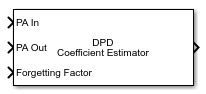Main Content

# DPD Coefficient Estimator

Estimate memory-polynomial coefficients for digital predistortion

• Library:
• Communications Toolbox / RF Impairments Correction

•## Description

Estimate memory-polynomial coefficients for digital predistortion (DPD) of a nonlinear power amplifier.

This icon shows the block with all ports enabled.## Ports

### Input

expand all

Power amplifier baseband-equivalent input, specified as a column vector.

Data Types: `double`
Complex Number Support: Yes

Power amplifier baseband-equivalent output, specified as a column vector of the same length as PA In.

Data Types: `double`
Complex Number Support: Yes

Forgetting factor used by the recursive least squares algorithm, specified as a scalar in the range (0, 1]. Decreasing the forgetting factor reduces the convergence time but causes the output estimates to be less stable.

#### Dependencies

To enable this port, set Algorithm to ```Recursive least squares``` and set Forgetting factor source to `Input port`.

Data Types: `double`

### Output

expand all

Memory-polynomial coefficients, returned as a matrix. For more information, see Digital Predistortion.

## Parameters

expand all

Desired amplitude gain in dB, specified as a scalar. This parameter value expresses the desired signal gain at the compensated amplifier output.

Tunable: Yes

Data Types: `double`

Polynomial type used for predistortion, specified as one of these values:

• `Memory polynomial` — Computes predistortion coefficients by using a memory polynomial without cross terms

• `Cross-term memory polynomial` — Computes predistortion coefficients by using a memory polynomial with cross terms

For more information, see Digital Predistortion.

Memory-polynomial degree, specified as a positive integer.

Data Types: `double`

Memory-polynomial depth in samples, specified as a positive integer.

Data Types: `double`

Adaptive algorithm used for equalization, specified as one of these values:

• `Least squares` — Estimate the memory-polynomial coefficients by using a least squares algorithm

• `Recursive least squares` — Estimate the memory-polynomial coefficients by using a recursive least squares algorithm

For algorithm reference material, see the works listed in  and .

Data Types: `char` | `string`

Source of the forgetting factor, specified as one of these values:

• `Property` — Specify this value to use the Forgetting factor parameter to specify the forgetting factor.

• `Input port` — Specify this value to use the Forgetting Factor input port to specify the forgetting factor.

#### Dependencies

To enable this parameter, set Algorithm to ```Recursive least squares```.

Data Types: `double`

Forgetting factor used by the recursive least squares algorithm, specified as a scalar in the range (0, 1]. Decreasing the forgetting factor reduces the convergence time but causes the output estimates to be less stable.

#### Dependencies

To enable this parameter, set Algorithm to ```Recursive least squares``` and set Forgetting factor source to `Property`.

Data Types: `double`

Initial coefficient estimate for the recursive least squares algorithm, specified as a matrix.

• If you specify this value as an empty matrix, the initial coefficient estimate for the recursive least squares algorithm is chosen automatically to correspond to a memory polynomial that is an identity function, so that the output is equal to input.

• If you specify this value as a nonempty matrix, the number of rows must be equal to the Memory depth parameter value.

• If the Polynomial type parameter is set to `Memory polynomial`, the number of columns is the degree of the memory polynomial.

• If the Polynomial type parameter is set to `Cross-term memory polynomial`, the number of columns must equal m(n-1)+1. m is the memory depth of the polynomial, and n is the degree of the memory polynomial.

For more information, see Digital Predistortion.

#### Dependencies

To enable this parameter, set Algorithm to ```Recursive least squares```.

Data Types: `double`
Complex Number Support: Yes

Type of simulation to run, specified as `Code generation` or `Interpreted execution`.

• `Code generation` –– Simulate the model by using generated C code. The first time you run a simulation, Simulink® generates C code for the block. The C code is reused for subsequent simulations unless the model changes. This option requires additional startup time, but the speed of the subsequent simulations is faster than `Interpreted execution`.

• `Interpreted execution` –– Simulate the model by using the MATLAB® interpreter. This option requires less startup time than the `Code generation` method, but the speed of subsequent simulations is slower. In this mode, you can debug the source code of the block.

## Block Characteristics

 Data Types `double` | `single` Multidimensional Signals `no` Variable-Size Signals `yes`

## More About

expand all

 Morgan, Dennis R., Zhengxiang Ma, Jaehyeong Kim, Michael G. Zierdt, and John Pastalan. "A Generalized Memory Polynomial Model for Digital Predistortion of Power Amplifiers." IEEE® Transactions on Signal Processing. Vol. 54, Number 10, October 2006, pp. 3852–3860.

 M. Schetzen. The Volterra and Wiener Theories of Nonlinear Systems. New York: Wiley, 1980.

## Support

#### Bridging Wireless Communications Design and Testing with MATLAB

Download white paper